# Electronics and Communication Engineering - Networks Analysis and Synthesis

1.

The relation AD - BC = 1 where A, B, C, D are transmission parameters of a network is valid for

 A. every network B. passive but not reciprocal network C. passive and reciprocal network D. both active and passive networks

Explanation:

No answer description available for this question. Let us discuss.

2.

In figure the phase plot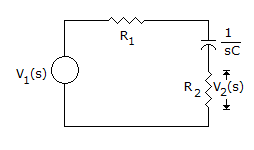A. will be 90° at ω = 0 and 0° at ω = ∞ B. will be 0° at ω = 0 and 90° at ω = ∞ C. will be 45° at ω = 0 and 0° at ω = ∞ D. will be 45° at ω = 0 and 90° at ω = ∞

Explanation:

No answer description available for this question. Let us discuss.

3.

The graph of a network is shown in the figure. Which one of the figure shown is not a tree of the graph?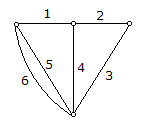A.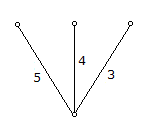B.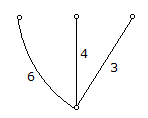C.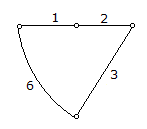D.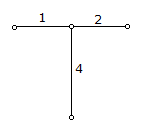Explanation:

No answer description available for this question. Let us discuss.

4.

A network N is dual of network N if

 A. both of them have same mesh equation B. both of them have same node equation C. mesh equation of one are the node equations of the other D. KCL and KVL equations are the same

Explanation:

No answer description available for this question. Let us discuss.

5.

The resistance of the aluminium conductor (r = 2.83 x 10-8 Ωm) in figure is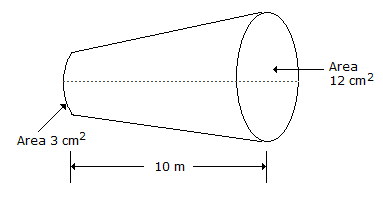A. 0.001 Ω B. less than 0.001 Ω C. more than 0.001 Ω D. more than 0.002 Ω